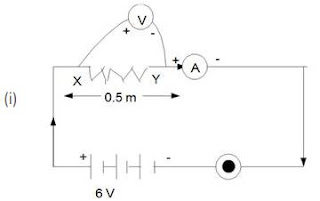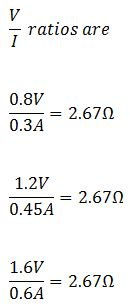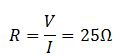## Solutions of Electricity Lakhmir Singh Manjit Kaur MCQ, HOTS, SAQ and VSAQ Pg No. 12 Class 10 Physics27. The other name of potential difference is :
A. ampereage
B. wattage
C. voltage
D. potential energy

→ Electric potential difference is known as voltage, which is equal to the work done per unit charge to move the charge between two points against static electric field. It is denoted by ‘V’.

28. Which statement/statements is/are correct?
1) An ammeter is connected in series in a circuit and a voltmeter is connected in parallel.
2) An ammeter has a high resistance.
3) A voltmeter has a low resistance.
A. 1, 2, 3
B. 1, 2
C. 2, 3
D. 1

→ An ammeter has to measure to current flowing through the circuit. Resistance offers an obstruction to the current flow. So, if the resistance of an ammeter is large, the current measured by the ammeter will be quite less as compared to the actual amount of current flowing through the circuit which is undesirable. If ammeter has zero resistance, then it will give the exact value of current. But this is not practically possible because every material has some value of internal resistan.

28. Which statement/statements is/are correct?
1) An ammeter is connected in series in a circuit and a voltmeter is connected in parallel.

2) An ammeter has a high resistance.
3) A voltmeter has a low resistance.
A. 1, 2, 3
B. 1, 2
C. 2, 3
D. 1

→ An ammeter has to measure to current flowing through the circuit. Resistance offers an obstruction to the current flow. So, if the resistance of an ammeter is large, the current measured by the ammeter will be quite less as compared to the actual amount of current flowing through the circuit which is undesirable. If ammeter has zero resistance, then it will give the exact value of current. But this is not practically possible because every material has some value of internal resistance which we can't control. For this reason, ammeter must have small resistance.

29. Which unit could be used to measure current?
A. Watt
B. Coulomb
C. Volt
D. Ampere

→ SI unit of electric current is ampere (A).

30. If the current through a flood lamp is 5 A, what charge passes in 10 seconds?
A. 0.5 C
B. 2 C
C. 5 C
D. 50 C

→ Charge = Current × time = 10 × 5 = 50

31. If the amount of electric charge passing through a conductor in 10 minutes is 300 C, the current flowing is :
A. 30 A
B. 0.3 A
C. 0.5 A
D. 5 A

→ Charge = Current × time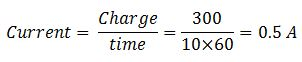Questions Based on High Order Thinking Skills (HOTS)-Pg-12

32. A student made an electric circuit shown here to measure the current through two lamps.
A. Are the lamps in series or parallel?
B. The student has made a mistake in this circuit.
What is the mistake?
C. Draw a circuit diagram to show the correct way to connect the circuit.
Use the proper circuit symbols in your diagram.

→ (a) The lamps are connected in series. In a series circuit, every device must function for the circuit to be complete.
(b) Here the students connected Ammeter in parallel with the lamps. An ammeter is always connected in series.

33. Draw a circuit diagram to show how 3 bulbs can be lit from a battery so that 2 bulbs are controlled by the same switch while the third bulb has its own switch.→ Two bulbs are connected in series, so that both bulbs are controlled by same switch and the third bulb is connected in parallel, so that it can be controlled by another switch.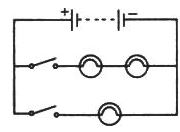34. An electric heater is connected to the 230 V mains supply. A current of 8A flows through the heater.
(a) How much charge flows around the circuit each second?
(b) How much energy is transferred to the heater each second?

→ (a) Here Potential difference = 230 V
Current = 8A
Time = 1 Second
As we know that
The flow of electric charge per unit time is known as electric current.So in each second the amount of charge flowing in the circuit is 8 C.
(b) As we know that the work done is equal to the amount of energy transferred in a circuit.
Electric potential difference is known as voltage, which is equal to the work done per unit charge to move the charge between two points against static electric field.So, Work done = 230 × 8 = 1840 J
It is also equal to the total energy transferred to the heater in each second.

35. How many electrons are flowing per second past a point in a circuit in which there is a current of 5 amp ?

→ Here
Q = I × t
As we know that
Q = ne
Ne = I × t1. Name the law which relates the current in a conductor to the potential difference across its ends.

→ The law stating that the direct current flowing in a conductor is directly proportional to the potential difference between its ends. It is usually formulated as V = IR. This law is called Ohm's law.

2. Name the unit of electrical resistance and give its symbol.

→ The ohm (symbol: Ω) is the SI derived unit of electrical resistance, named after German physicist Georg Simon Ohm.

3. Name the physical quantity whose unit is "ohm".

→ The ohm (symbol: Ω) is the SI derived unit of electrical resistance.

4. What is the general name of the substances having infinitely high electrical resistance?

→ Substances having infinite resistivity are called insulators Ex: wood.

5. Keeping the resistance constant, the potential difference applied across the ends of a component is halved.
By how much does the current change?

→ As we know thatAs Current is directly proportional to potential difference.
So Current is also become half.

6. State the factors on which the strength of electric current flowing in a given conductor depends.

→ The strength of electric current flowing in a given conductor depends upon the potential difference and resistance of the conductor.

7. Which has less electrical resistance: a thin wire or a thick wire (of the same length and same material) ?

→ The larger the cross sectional area, the lower the resistance since the electrons have a larger area to flow through.

8. Keeping the potential difference constant, the resistance of a circuit is halved. By how much does the current change?

→ As we know that
V = IR
As current is directly proportional to potential difference and inversely proportional to the resistance of the conductor. If resistance of a circuit is halved than the current will doubled.

9. A potential difference of 20 volts is applied across the ends of a resistance of 5 ohms. What current will flow in the resistance?

→ As we know that10. A resistance of 20 ohms has a current of 2 amperes flowing in it. What potential difference is there between its ends?

→ As we know that
V = IR
V = 2A × 20 ohms = 40 V.

11. A current of 5 amperes flows through a wire whose ends are at a potential difference of 3 volts. Calculate the resistance of the wire.

→ As we know that
V = IR12. Fill in the following blank with a suitable word :
Ohm's law states a relation between potential difference and ........................

→ Ohm's law states a relation between potential difference and current.

13. Distinguish between good conductors, resistors and insulators. Name two good conductors, two resistors and two insulators.

→ On the basis of their electrical resistance, all the substance can be divided into three groups they are Good conductors, Resistors and Insulators.
Those substances which have very low electrical resistance are called as good conductors. A good conductor allows the electricity to flow through it easily. Silver is the best conductor of electricity. Copper and aluminum are also good conductors.
Those substances which have comparatively high resistance than conductors are known as resistors. The alloys like nichrome, manganin and constantan, all have quite high resistance, so these are resistors.
Those substances which have infinitely high electrical resistance are called insulators. E.g- rubber and wood.

14. Classify the following into good conductors, resistors and insulators:
Rubber, Mercury, Nichrome, Polythene, Aluminium, Wood, Manganin, Bakelite, Iron, Paper, Thermocol, Metal coin.

→ Those substances which have very low electrical resistance are called as good conductors like mercury, aluminum, iron, metal coin.
Those substances which have comparatively high resistance than conductors are known as resistors. The alloys like nichrome, manganin.
Those substances which have infinitely high electrical resistance are called insulators like rubber, polythene, wood, bakelite, paper, thermocol

15. What is Ohm's law? Explain how it is used to define the unit of resistance.

→ Ohm's law states that the current through a conductor between two points is directly proportional to the voltage across the two points. Introducing the constant of proportionality, the resistance, one arrives at the usual mathematical equation that describes this relationship
I = V R
Where I is the current through the conductor in units of amperes, V is the voltage measured across the conductor in units of volts, and R is the resistance of the conductor in units of ohms. More specifically, Ohm's law states that the R in this relation is constant, independent of the current.
One ohm is defined as the resistance of an object when a current of one ampere flows through a an object with a potential difference of one volt.

16A. What is meant by the "resistance of a conductor"? Write the relation between resistance, potential difference and current.

→ The resistance of a conductor is the ratio of potential difference between the ends of a conductor to the current flowing through it, or the property of a conductor due to which it opposes the flow of current through it is called resistance of the conductor.
Potential differences(V) = Current (I) × Resistance(R).

16B. When a 12 V battery is connected across an unknown resistor, there is a current of 2.5 mA in the circuit. Calculate the value of the resistance of the resistor.

→ According to Question,
V = 12volt I = 2.5 mA= 2.5x10-3A
According to Ohms Law
V=IR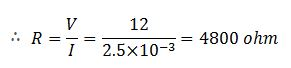17A. Define the of resistance (or Define the unit "ohm").

→ The ohm is defined as a resistance between two points of a conductor when a constant potential difference of 1.0 volt, applied to these points, produces in the conductor a current of 1.0 ampere, the conductor not being the seat of any electromotive force.

17B. What happens to the resistance as the conductor is made thinner?

→ The resistance of the wire will increase if we made the conductor thinner because p (rho).. Area is directly proportional to the radius of the wire.

17C. Keeping the potential difference constant, the resistance of a circuit is doubled. By how much does the current changes?

→ As we know that
V = IR
So, I = V/R
According to the questions
V= I'2R
So, I'= V/2R
I' = I/2
So current become half.

18A. Why do electricians wear rubber hand gloves while working with electricity ?

→ Rubber is an insulator and does not allow the passage of electric current through it, so when electricians wear rubber gloves electric current cannot pass through them, so they don't get shock.

18B. What p.d. is needed to send a current of 6 A through an electrical appliance having a resistance of 40 Ω

→ As we know that
V = IR
Here I = 6A, R = 40Ω
So V = 6 × 40 = 240V

19. An electric circuit consisting of a 0.5 m long nichrome wire XY, an ammeter, a voltmeter, four cells of 1.5 V each and a plug key was set up.
(i) Draw a diagram of this electric circuit to study the relation between the potential difference maintained between the points 'X' and 'Y' and the electric current flowing through XY.
(ii) Following graph was plotted between V and I values:
What would be the value of ratios when the potential differences us 0.8 V, 1.2 V and 1.6 V respectively?
What conclusion does you drawn from the values?
(iii) What is the resistance of the wire?